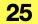Describes the Structural Analytical Model Analytical Surface Load settings.

```    typedef struct {
API_AnalyticalCoordSysDirection         coordinateSystemType;
double                                  xValue;
double                                  yValue;
double                                  zValue;
```

#### Members

loadCaseIdThe guid of the connected Load Case.
coordinateSystemTypeDefines the co-ordinate system of the member in which the load is applied. For ” Local”, coordinate system is defined by the member where is load applied
xValueThe force/moment value in X direction of the Analytical Surface Load
yValueThe force/moment value in Y direction of the Analytical Surface Load
zValueThe force/moment value in Z direction of the Analytical Surface Load

#### Requirements

Version: API 25 or later